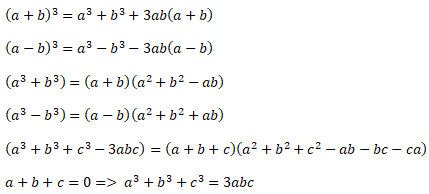# ALL ALGEBRA Maths Formulas and theorems

## ALL ALGEBRA FORMULAS### Factor Theorem:

If (x-a) is the factor of f(x), then f (a) =0

### Remainder Theorem:

If f(x) is divided by (x-a), then Remainder =f (a)

### Some special resultswill always be completely divisible by ( x+a), While n is an even number.will always be completely divisible by ( x+a), While n is an odd number.will never be completely divisible by (x-a).

### SURDS

Let ‘a’ is a rational number. And ‘n’ is a positive integer. Thenis called Surds of nth Power, while nth Power of any positive rational number is not equal to ‘a’.

Note: It is not necessary to have every irrational number a surd.

### Pure Surd:

If any monolithic has coefficient 1. Then this called Pure Surd.

### Mixed Surd:

If a monolithic has any rational coefficient other than 1, then it’s called Mixed Surd.

### Degree of Polynomial:

The exponent of the term with the largest degree in a polynomial is called the power of that polynomial.

### Linear Polynomial:

If the maximum power of the variable used in the polynomial is 1, it is called linear polynomial.